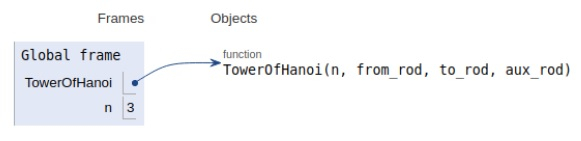# Python Program for Tower of Hanoi

Problem statement − We are given n disks and a series of rods, we need to transfer all the disks to the final rod under the given constraints−

• We can move only one disk at a time.

• Only the uppermost disk from the rod can be moved.

• Any bigger disk cannot be placed on the smaller disk

Now let’s observe the solution in the implementation below −

## Example

Live Demo

# tower of hanoi
def TowerOfHanoi(n , from_rod, to_rod, aux_rod):
if n == 1:
print ("Move disk 1 from rod",from_rod,"to rod",to_rod)
return
TowerOfHanoi(n-1, from_rod, aux_rod, to_rod)
print ("Move disk",n,"from rod",from_rod,"to rod",to_rod)
TowerOfHanoi(n-1, aux_rod, to_rod, from_rod)
# main
n = 3
TowerOfHanoi(n, 'A', 'C', 'B')
# A, B, C are the rod
print ("Sorted array is:")
for i in range(n):
print (arr[i],end=" ")

## Output

Move disk 1 from rod A to rod C
Move disk 2 from rod A to rod B
Move disk 1 from rod C to rod B
Move disk 3 from rod A to rod C
Move disk 1 from rod B to rod A
Move disk 2 from rod B to rod C
Move disk 1 from rod A to rod CAll the variables are declared in the local scope and their references are seen in the figure above.

## Conclusion

In this article, we have learned about how we can make a Python Program for Tower of Hanoi